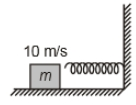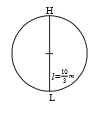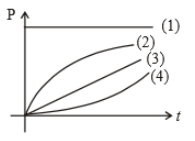Select Question Set:# A block of mass 2 kg moving with velocity of 10 m/s on a smooth surface hits a spring of force constant $80×{10}^{3}$ N/m as shown. The maximum compression in the spring is1. 5 cm 2. 10 cm 3. 15 cm 4. 20 cmSubtopic:  Elastic Potential Energy |
70%
From NCERT
To view explanation, please take trial in the course.
NEET 2023 - Target Batch - Aryan Raj Singh
Hints
To view explanation, please take trial in the course.
NEET 2023 - Target Batch - Aryan Raj Singh

A body starts moving from rest in straight line under a constant power source. Its displacement in time t is proportional to

(1) ${t}^{1/2}$

(2) t

(3) ${t}^{3/2}$

(4) ${t}^{2}$Subtopic:  Power |
58%
From NCERT
To view explanation, please take trial in the course.
NEET 2023 - Target Batch - Aryan Raj Singh
Hints
To view explanation, please take trial in the course.
NEET 2023 - Target Batch - Aryan Raj Singh

A block of mass m = 25 kg on a smooth horizontal surface with a velocity $\stackrel{\to }{v}$ =3 $m{s}^{-1}$ meets the spring of spring constant k = 100 N/m fixed at one end as shown in the figure. The maximum compression of the spring and velocity of the block as it returns to the original position respectively are:1.  1.5 m, -3 $m{s}^{-1}$

2.  1.5 m, 0 $m{s}^{-1}$

3.  1.0 m, 3 $m{s}^{-1}$

4.  0.5 m, 2 $m{s}^{-1}$Subtopic:  Elastic Potential Energy |
73%
From NCERT
To view explanation, please take trial in the course.
NEET 2023 - Target Batch - Aryan Raj Singh
Hints
To view explanation, please take trial in the course.
NEET 2023 - Target Batch - Aryan Raj Singh

The velocity, given to the block of mass (m), is to rotate it in a circle of radius l. Calculate the height (h) where the block leaves the circle.

1. $\frac{3l}{2}$

2. $\frac{4l}{3}$

3. $\frac{5l}{4}$

4. None of theseSubtopic:  Work Energy Theorem |
From NCERT
To view explanation, please take trial in the course.
NEET 2023 - Target Batch - Aryan Raj Singh
Hints
To view explanation, please take trial in the course.
NEET 2023 - Target Batch - Aryan Raj SinghIf length of string is

$\mathrm{where}$

1.  10 m/s

2.  20 m/s

3.  $\frac{10}{\sqrt{2}}\mathrm{m}/\mathrm{s}$

4.Subtopic:  Work Energy Theorem |
From NCERT
To view explanation, please take trial in the course.
NEET 2023 - Target Batch - Aryan Raj Singh
Hints
To view explanation, please take trial in the course.
NEET 2023 - Target Batch - Aryan Raj Singh

The relation between velocity (v) and time (t) is $v\propto \sqrt{\mathrm{t}}$, then which one of the following quantity is constant?

1.  Force

2.  Power

3.  Momentum

4.  Kinetic EnergySubtopic:  Power |
61%
From NCERT
To view explanation, please take trial in the course.
NEET 2023 - Target Batch - Aryan Raj Singh
Hints
To view explanation, please take trial in the course.
NEET 2023 - Target Batch - Aryan Raj Singh

A particle is moving on the circular path of the radius (R) with centripetal acceleration ${a}_{c}={k}^{2}R{t}^{2}$. Then the correct relation showing power (P) delivered by net force versus time (t) is1. 1

2. 2

3. 3

4. 4Subtopic:  Power |
To view explanation, please take trial in the course.
NEET 2023 - Target Batch - Aryan Raj Singh
Hints

A steel wire can withstand a load up to 2940 N. A load of 150 kg is suspended from a rigid support. The maximum angle through which the wire can be displaced from the mean position, so that the wire does not break when the load passes through the position of equilibrium, is (2008 E)

1. 30$°$

2. 60$°$

3. 80$°$

4. 85$°$Subtopic:  Work Energy Theorem |
68%
From NCERT
To view explanation, please take trial in the course.
NEET 2023 - Target Batch - Aryan Raj Singh
Hints
To view explanation, please take trial in the course.
NEET 2023 - Target Batch - Aryan Raj Singh

A sphere of mass m moving with constant velocity hits another sphere of the same mass at rest. If e is the coefficient of restitution. The ratio of their velocities after the collision is

1.  1 + e

2.

3.

4.Subtopic:  Collisions |
75%
To view explanation, please take trial in the course.
NEET 2023 - Target Batch - Aryan Raj Singh
Hints

A body is thrown vertically up with a certain initial velocity. The potential and the kinetic energy of the body are equal at a point P in its path. If the same body is thrown with double the velocity upwards, the ratio of the potential and the kinetic energies of the body when it crosses at the same point will be:

1. 1:1

2. 1:4

3. 1:7

4. 1:8Subtopic:  Conservation of Mechanical Energy |
From NCERT
To view explanation, please take trial in the course.
NEET 2023 - Target Batch - Aryan Raj Singh
Hints